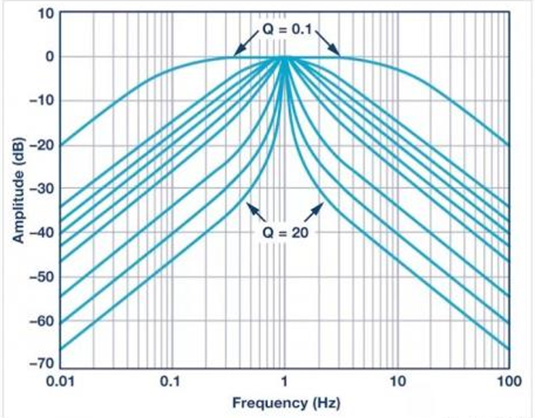# The importance of dB for RF design

In the face of a project indicator of RF design, one of the most common words is “dB”. For an RF engineer, dB is sometimes as familiar as its name. dB is a logarithmic unit that provides a convenient way to express ratios, such as the ratio between an input signal and an output signal.

Since dB is a ratio, it is a relative unit, not absolute. The voltage of the signal is measured absolutely, because we always say potential difference, that is, the potential difference between two points; Usually we refer to the potential of a node relative to the 0 V ground node. The current of the signal is also measured absolutely, since the unit (ampere) involves a specific amount of charge for a specific amount of time. In contrast, dB is a unit that involves the logarithm of the ratio between two numbers. For example, amplifier gain: If the power of the input signal is 1 W and the power of the output signal is 5 W, the ratio is 5, which converts to dB is 6.9897dB.

Therefore, the amplifier provides a power gain of 7dB, that is, the ratio between the output signal strength and the input signal strength can be expressed as 7dB.

Why use dB?

It is certainly possible to design and test RF systems without using dB, but in reality, dB is ubiquitous. One advantage is that the dB scale allows us to express very large ratios without using very large numbers: 1,000,000 has a power gain of only 60dB. In addition, the total gain or loss of the signal chain is in the dB domain and is easy to calculate because the individual dB numbers are simply added (whereas if we use ordinary ratios, multiplication is required).

Another advantage is what we are familiar with from the experience of filters. RF systems revolve around frequencies and the various ways in which frequencies are generated, controlled, or affected by components and parasitic circuit components. The dB scale is convenient in such a context because the frequency response plot is intuitive and visually informative when the frequency axis uses the logarithmic scale and the amplitude axis uses the dB scale.Therefore, in the process of designing the filter, it is necessary to be quite careful.